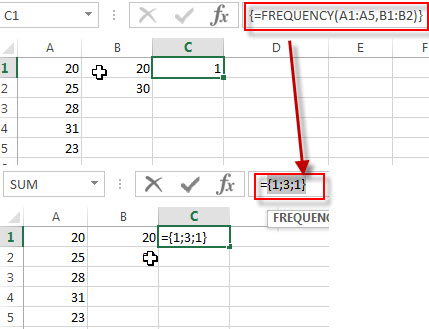## How to Count Unique Numeric Values with Criteria in a Range in Excel

We have talked that how to count unique numeric values in a data set in Excel in the previous post. And this post will guide you how to count unique numeric values with criteria in the range in Excel 2013/2016 or Excel office 365. How do I count the unique numeric values with a criteria in a list of data with some duplicate values using a formula in Excel. And you can use a formula that uses the SUM function, the FREQUENCY function and the IF function to count unique values in a range based on a criteria in Excel.

## COUNT Unique Numeric Values with Criteria

Assuming you have a list of data range A1:B6 in your worksheet and you wish to count the number of unique score numbers in grade “A” using a formula. And the below introduction will show you the ways to achieve the result. The generic formula is like this:

=SUM(–(FREQUENCY(IF(B2:B7=”A”,A2:A7),A2:A7)>0))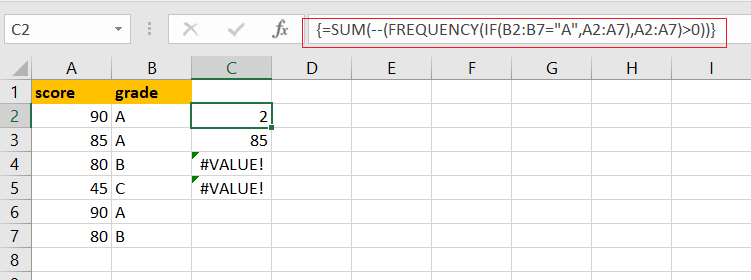Note: if you are working in Excel 365 version, and you can use a dedicated function called UNIQUE to count unique values. It can be used to count unique values including numeric or text values.

Let’s See How This Formula Works:

=IF(B2:B7=”A”,A2:A7)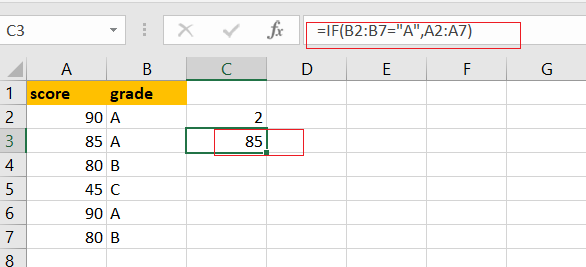You can use the IF function to filter the value in column B if the grade value in Column B is “A”, then returns the value in Column A. and The result is in an array like this:

{90;85;FALSE;FALSE;90;FALSE}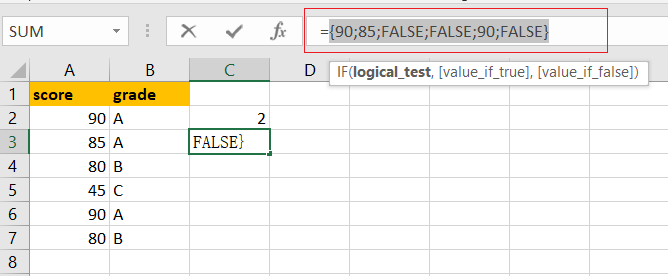You can notice that if the value is not grade “A”, and it will return the FALSE value. And this array is passed to the FREQUENCY function as the argument.

= FREQUENCY(IF(B2:B7=”A”,A2:A7),A2:A7)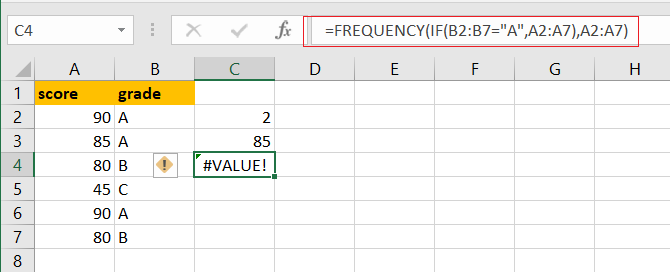The FREQUENCY function can be used to count each of numeric value in the above array list, and returns an array result, like this:

{2;1;0;0;0;0;0}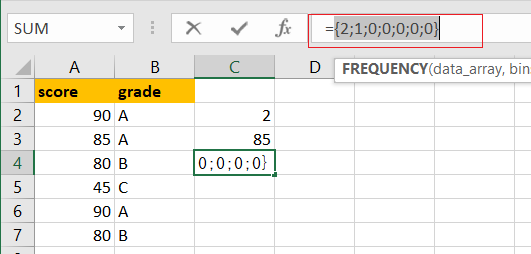From the above array result, you can see that the FREQUENCY function returns zero for any numbers that appear more that once in the data array, which is why values are zero once a number has been counted.

=FREQUENCY(IF(B2:B7=”A”,A2:A7),A2:A7)>0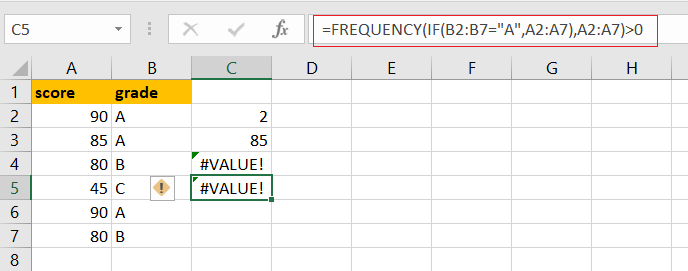You need to test each values that is greater than zero. The array result is like this:

{TRUE;TRUE;FALSE;FALSE;FALSE;FALSE;FALSE}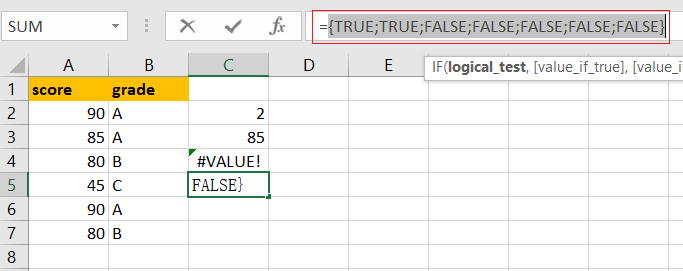Echo “TRUE” value represents a unique numeric value in the range A2:A7, and you still need to add up the TRUE values with the SUM function.

=–(FREQUENCY(IF(B2:B7=”A”,A2:A7),A2:A7)>0)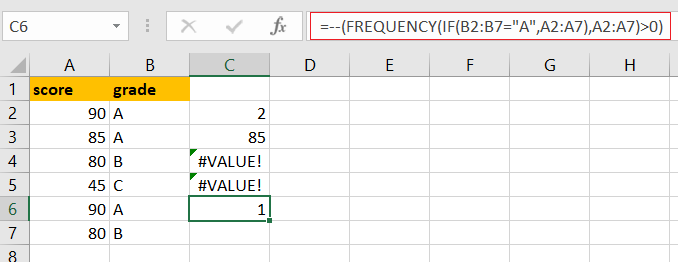The SUM function can be used to add up logic values in an array, and you need to coerce those values into 1 or 0 by the double negative operator

{1;1;0;0;0;0;0}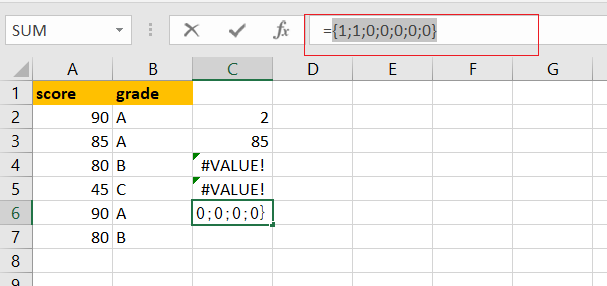Finally, you can use the SUM function to adds these values up in the above result array and press “CTRL+Shift+Enter” keys to change this normal formula as an array formula, and return the total number is 2.

### Related Functions

• Excel SUM function
The Excel SUM function will adds all numbers in a range of cells and returns the sum of these values. You can add individual values, cell references or ranges in excel.The syntax of the SUM function is as below:= SUM(number1,[number2],…)…
• Excel FREQUENCY function
TThe Excel FREQUENCY function calculates how often values occur within a range of values. And it will return a vertical array of numbers.The syntax of the FREQUENCY function is as below:= FREQUENCY (data_array, bins_array)…
• Excel IF function
The Excel IF function perform a logical test to return one value if the condition is TRUE and return another value if the condition is FALSE. The IF function is a build-in function in Microsoft Excel and it is categorized as a Logical Function.The syntax of the IF function is as below:= IF (condition, [true_value], [false_value])….

## How to Count Unique Numeric Values in a Range in Excel

This post will guide you how to count unique numeric values in the given range in Excel 2013/2016 or Excel office 365. How do I count the unique numeric values in a list of data with some duplicate values using a formula in Excel. And you can use a formula that uses the SUM function together with the FREQUENCY function to count unique values in a range.

## COUNT Unique Numeric Values

Assuming you have a list of data range B1:B6 in your worksheet and you wish to count the number of unique numeric values using a formula. And the below introduction will show you the ways to achieve the result. The generic formula is like this:

=SUM(–(FREQUENCY(B1:B6,B1:B6)>0))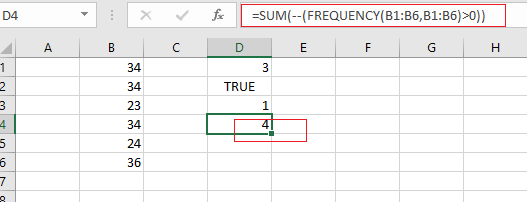Note: if you are working in Excel 365 version, and you can use a dedicated function called UNIQUE to count unique values. It can be used to count unique values including numeric or text values.

Let’s See How This Formula Works:

=FREUQENCY(B1:B6,B1:B6)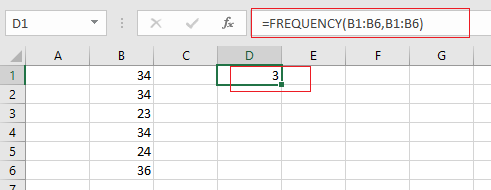The FREQUENCY function can be used to count each of numeric value in the range B1:B6, and returns an array result, like this:

{3;0;1;0;1;1;0}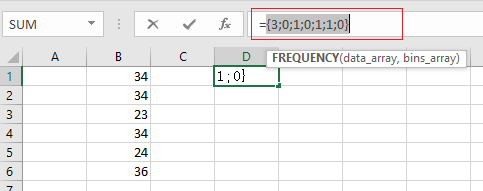From the above array result, you can see that the FREQUENCY function returns zero for any numbers that appear more that once in the data array, which is why values are zero once a number has been counted.

= FREQUENCY(B1:B6,B1:B6)>0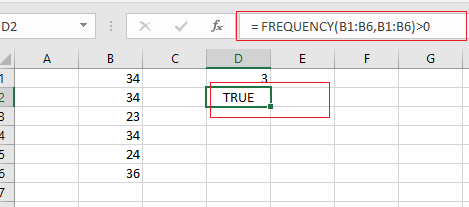You need to test each values that is greater than zero. The array result is like this:

{TRUE;FALSE;TRUE;FALSE;TRUE;TRUE;FALSE}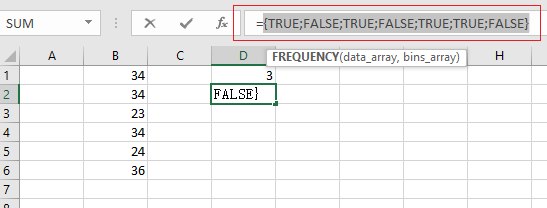Echo “TRUE” value represents a unique numeric value in the range B1:B6, and you still need to add up the TRUE values with the SUM function.

= –(FREQUENCY(B1:B6,B1:B6)>0)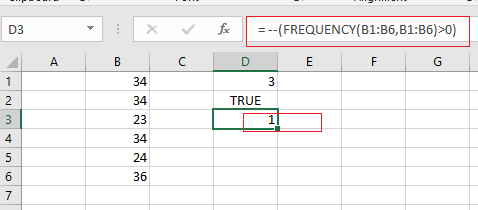The SUM function can be used to add up logic values in an array, and you need to coerce those values into 1 or 0 by the double negative operator

{1;0;1;0;1;1;0}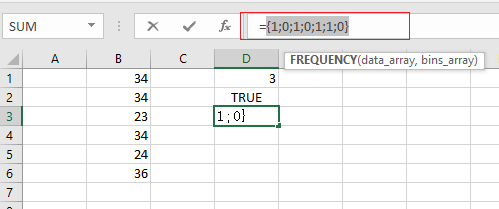Finally, you can use the SUM function to adds these values up in the above result array and return the total number is 4. Also you can use the SUMPRODUCT function to sum the values in the given array.

You can also use another way to count unique numeric values that is to use the COUNTIF function on the larger data list.

### Related Functions

• Excel SUM function
The Excel SUM function will adds all numbers in a range of cells and returns the sum of these values. You can add individual values, cell references or ranges in excel.The syntax of the SUM function is as below:= SUM(number1,[number2],…)…
• Excel FREQUENCY function
TThe Excel FREQUENCY function calculates how often values occur within a range of values. And it will return a vertical array of numbers.The syntax of the FREQUENCY function is as below:= FREQUENCY (data_array, bins_array)…
• Excel SUMPRODUCT function
The Excel SUMPRODUCT function multiplies corresponding components in the given one or more arrays or ranges, and returns the sum of those products.The syntax of the SUMPRODUCT function is as below:= SUMPRODUCT (array1,[array2],…)…
• Excel COUNTIF function
The Excel COUNTIF function will count the number of cells in a range that meet a given criteria. This function can be used to count the different kinds of cells with number, date, text values, blank, non-blanks, or containing specific characters.etc.= COUNTIF (range, criteria)…

## How to Count Unique Dates in Excel

This post will guide you how to count unique dates in a given range in Excel 2013/2016 or Excel office 365. How do I count the unique dates in a list of dates with some duplicate dates using a formula in Excel. And you can do this by a formula based on the SUMPRODUCT function and the COUNTIF function in an older version of Excel 2013/2016. You can also do it using UNIQUE function in the latest Excel 365.

Assuming you have a list of data range B1:B6 in your worksheet and you wish to count the number of unique dates using a formula. And the below introduction will show you the ways to achieve the result.

## Count Unique Dates in Old Version of Excel

If you are using older version of Excel, for example, Excel 2013 or Excel 2016, and you can use the COUNTIF function in combination with the SUMPRODUCT function to count unique dates with a formula like this:

`=SUMPRODUCT(1/COUNTIF(B1:B6,B1:B6))`

Let’s See How This Formula Works:

`=COUNTIF(B1:B6,B1:B6)`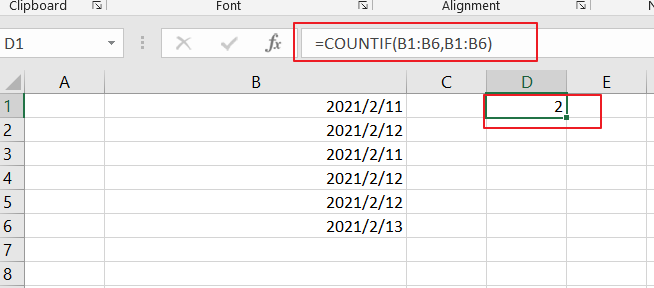The COUNTIF function will compare each date in the same data range and returns an array results that contain a count for every data in the given range. The result array is like this:

`{2;3;2;3;3;1}`
`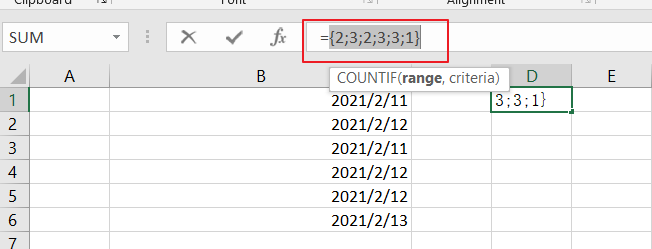`

Then you need to divide this array result by number 1 to return an array of fractional values like this:

`=1/COUNTIF(B1:B6,B1:B6)`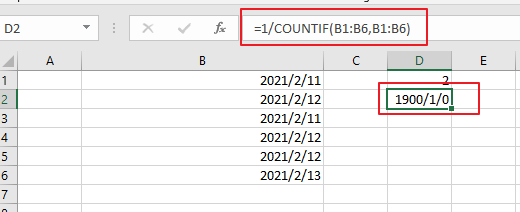`={0.5;0.333333333333333;0.5;0.333333333333333;0.333333333333333;1}`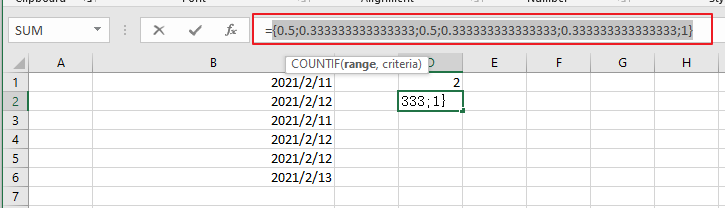Finally, you still need to add up the items in the above array. And you can use the SUMPRODUCT function to sum up the items in that array list. And it will return the total unique dates as 3.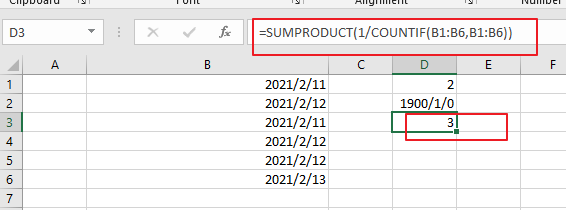You can also use another array formula based on the SUM Function and the FREQUENCY function to achieve the result of counting unique dates in the given date range. Like below:

`=SUM(--(FREQUENCY(B1:B6,B1:B6)>0))`

Then you need to put this formula into a blank cell and press “Ctrl +Shift +Enter” to make it as array formula.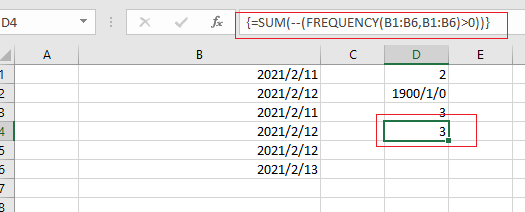Count Unique Dates in Excel 365

If you are working in Excel 365 and you can use the UNIQUE function in combination with the COUNT function to count unique dates in the given range cells in Excel.

The generic formula is like this:

`=COUNT(UNIQUE(B1:B6)`

The UNIQUE function can be used to extract a list of unique dates from a given range B1:B6, and then using the COUNT function to count numeric values. And if you wish to count both numeric and text values, you can replace the COUNT function with COUNTA function.

### Related Functions

• Excel SUMPRODUCT function
The Excel SUMPRODUCT function multiplies corresponding components in the given one or more arrays or ranges, and returns the sum of those products.The syntax of the SUMPRODUCT function is as below:= SUMPRODUCT (array1,[array2],…)…
• Excel COUNTIF function
The Excel COUNTIF function will count the number of cells in a range that meet a given criteria. This function can be used to count the different kinds of cells with number, date, text values, blank, non-blanks, or containing specific characters.etc.= COUNTIF (range, criteria)…
• Excel SUM function
The Excel SUM function will adds all numbers in a range of cells and returns the sum of these values. You can add individual values, cell references or ranges in excel.The syntax of the SUM function is as below:= SUM(number1,[number2],…)…
• Excel COUNT function
The Excel COUNT function counts the number of cells that contain numbers, and counts numbers within the list of arguments. It returns a numeric value that indicate the number of cells that contain numbers in a range…
• Excel FREQUENCY function
TThe Excel FREQUENCY function calculates how often values occur within a range of values. And it will return a vertical array of numbers.The syntax of the FREQUENCY function is as below:= FREQUENCY (data_array, bins_array)…
• Excel COUNTA function
The Excel COUNTA function counts the number of cells that are not empty in a range. The syntax of the COUNTA function is as below:= COUNTA(value1, [value2],…)…
• Excel UNIQUE function
The guide demonstrates how to use the UNIQUE function and dynamic arrays in Excel to create unique values.The syntax:=UNIQUE(array, [by col], [once exactly]) …

## Excel FREQUENCY Function

This post will guide you how to use the FREQUENCY function with syntax and examples in Microsoft excel.

### Description

The Excel FREQUENCY function calculates how often values occur within a range of values. And it will return a vertical array of numbers. For example, you can use the FREQUENCY function to get the number of scores that fall within ranges of scores.

The FREQUENCY function is a build-in function in Microsoft Excel and it is categorized as a Statistical Function.

The FREQUENCY function is available in Excel 2016, Excel 2013, Excel 2010, Excel 2007, Excel 2003, Excel XP, Excel 2000, Excel 2011 for Mac.

### Syntax

The syntax of the FREQUENCY function is as below:

`= FREQUENCY (data_array, bins_array)`

Where the FREQUENCY function arguments are:

• Data_array -This is a required argument.  An array or reference or range of values for which you want to count the frequencies. And if the data_array do not contain any values, then the FREQUENCY function will return an array of zeros.
• bins_array – This is a required argument.  An array or reference or range of values of intervals that you want to group the values in data_array. And if bins_array do not contain any values, the FREQUENCY function will return the number of elements in data_array.

Note:

• If you want to return array result, then you need to the FREQUENCY formula as Array formula. When you create array formula, you need to press Ctrl + Shift + Enter on the formula box. And you will see that it will create brackets {} around your formula. You should know that if there is no brackets around your formula, it will not be treated as an array formula.
• After you select a range of adjacent cells into which you want to return distribution, and the FREQUENCY need to be entered as an array formula.
• The FREQUENCY function will ignore blank cells and contain text.

### Excel FREQUENCY Function Examples

The below examples will show you how to use Excel FREQUENCY Function to get the frequency of values in a data set.

1# to count the frequency of values in a data set, with data_array A1:A5, and bins_array B1:B2 , using the following array formula (you must be entered as an array formula via pressing Ctrl + Shift + Enter shortcuts):

`{=FREQUENCY(A1:A5,B1:B2)}`